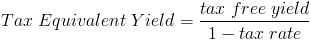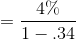# Tax Equivalent YieldThe tax equivalent yield formula is used to compare the yield between a tax-free investment and an investment that is taxed. One of the most common examples of a tax-free investment is municipal bonds. Municipal bonds are generally issued by local governments to finance development in its local community.

## Use of Tax Equivalent Yield Formula

Investors who pay higher taxes may want to consider investing in municipal bonds or equivalent investments. The higher tax bracket an individual is in, the more appealing a tax-free investment would become. The tax equivalent yield formula can be used to compare the yield on the tax-free investment vs. an investment that is taxed.

## Example of Tax Equivalent Yield

It is important to note before proceeding to this tax equivalent yield formula example that it is assumed that both bonds have equal risk. That is to say, that with the exception of the differences in yield, all things are held constant. Differences in risk do not affect the formula itself, but should be considered in any investment decision and not just the yield.

An example of the tax equivalent yield formula would be an investor who must decide between a bond that pays 6% that is taxed and a bond that pays 4% but earnings are tax free. His marginal tax rate is 34%. In order to compare the yield of these two investments, the equation for this example using the tax equivalent yield formula would beAfter solving the formula, the equivalent yield for 4% would be 6.06%. This rate is higher than the 6% rate from the bond that is taxed and will give a higher after-tax return.

New to Finance?